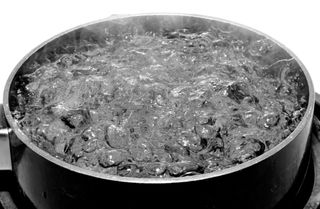# Fahrenheit: Facts, History & Conversion FormulasIn the Fahrenheit scale, water boils at 212 degrees.

Fahrenheit is a temperature scale that bases the boiling point of water at 212 and the freezing point at 32. It was developed by Daniel Gabriel Fahrenheit, a German-born scientist who lived and worked primarily in the Netherlands. Today, the scale is used primarily in the United States and some Caribbean countries. The rest of the world uses the Celsius scale.

In 1714, Fahrenheit developed the first modern thermometer — the mercury thermometer, with more refined measurements than previous temperature gauges. Fahrenheit’s thermometer was a take on an alcohol-based thermometer invented by Olaus Roemer, a Danish scientist. Roemer marked two points on his thermometer — 0 as the lowest point, 60 as the temperature of boiling water, 7.5 as the point where ice melted and 22.5 as body temperature.

Because the mercury thermometer was more accurate, Fahrenheit decided to expand the Roemer scale by multiplying its values by four. He made adjustments to those metrics based on further research, even putting the thermometer under his wife’s armpit to gain a body temperature.

In his initial scale, the zero point was determined by placing the thermometer in an equal mix of ice, water, and salt (ammonium chloride). This stable temperature was set as 0. The second point, at 32, was an equal mix of ice and water. The third point, 96, was approximately the human body temperature, referred to as "blood-heat."

While Fahrenheit documented in an article that he used the boiling and freezing points of water to build his scale, there were some conspiracy theorists who thought he had other motives for using those numbers. Some believe that Fahrenheit was a Freemason, and because there are 32 degrees of enlightenment, he chose to use 32 as the melting temperature of water. However, there is no record that the scientist was a Freemason.

In yet another story, it is said that Fahrenheit believed that a person would freeze to death at 0 degrees and would succumb to a heat stroke at 100.

The scale was recalibrated after his death, marking 32 and 212 as the exact melting and boiling points of plain water, minus the salt. It also makes the normal body temperature 98.6, which is what has become the standard.

That change made Celsius-to-Fahrenheit conversions easier. The size of the Fahrenheit "degree" is five-ninths the size of the same unit on Celsius and Kelvin temperature scales. This makes it easier to provide more exact measurements without using fractions in the Fahrenheit scale.

The Fahrenheit and Celsius scales converge at minus 40 F, so that minus 40 F and minus 40 C represent the same temperature.

## Who uses Fahrenheit?

Fahrenheit has widely been replaced by Celsius in most countries and for most applications. In the late 1960s and 1970s, the Celsius scale was phased in by governments around the world as part of the move to standardize on metric measurements.

Today, the scale is primarily used in the United States, and is also used in the Cayman Islands, Palau, Bahamas and Belize. While other branches of science use the Celsius scale, U.S. meteorologists continue to use the Fahrenheit scale for weather forecasting and reporting. Canadian meteorologists sometimes use the Fahrenheit scale alongside the Celsius scale.

Supporters of the Fahrenheit scale note that a degree on the Fahrenheit scale is the temperature change that the average person can detect.

## Conversion formulas

• Fahrenheit to Celsius: Subtract 32, then multiply by 5, then divide by 9
• Celsius to Fahrenheit: Multiply by 9, divide by 5, then add 32
• Fahrenheit to Kelvin: Subtract 32, multiply by 5, divide by 9, then add 273.15
• Kelvin to Fahrenheit: Subtract 273.15, multiply by 1.8, then add 32

Related: